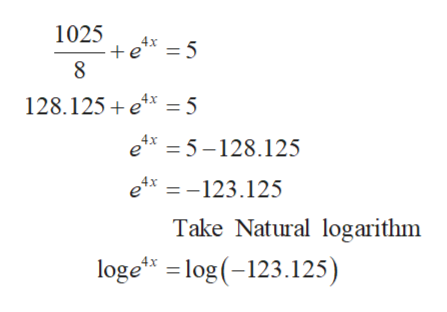Pre calcSolve the equation algebraically, round to 3 decimal place1025/8+e^4x=5Please show the steps and explain

Question

Pre calc

Solve the equation algebraically, round to 3 decimal place

1025/8+e^4x=5

Please show the steps and explain

Step 1

The given equation is,

Step 2

This can be simpl...help_outlineImage Transcriptionclose1025 5 128.125 ex 5 ex =5-128.125 ex123.125 Take Natural logarithm loge log (-123.125) fullscreen

Want to see the full answer?

See Solution

Want to see this answer and more?

Our solutions are written by experts, many with advanced degrees, and available 24/7

See Solution
Tagged in

Functions